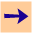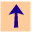1 Graph Searching and the Generic Search Algorithm

# 1 Graph Searching and the Generic Search Algorithm

Many AI problems can be cast as the problem of finding a path in a graph. A graph is made up of nodes and arcs. Arcs are ordered pairs of nodes that can have associated costs.

Suppose we have a set of nodes that we call "start nodes" and a set of nodes that we call "goal nodes", a solution is a path from a start node to a goal node.

Consider the following simple graph (this is a tree as there is at most one arc going into each node). The start nodes are colored grey, the goal nodes as are colored yellow, and the other nodes are not coloured.

To find a solution, we need to search for a path. We use the generic searching algorithm. The frontier is a set of paths from a start node (we often identify the path with the node at the end of the path). The nodes at the end of the frontier are outlined in green or blue. Initially the frontier is the set of empty paths from start nodes. Intuitively the generic graph searching algorithm is:

• Repeat
• select a path on the frontier. Let's call the path selected P.
• if P is a path to a goal node, stop and return P,
• remove P from the frontier
• for each neighbor of the node at the end of P, extend P to that neighbour and add the extended path to the frontier
• Until the frontier is empty. When it is empty there are no more solutions.

To see how this works you can carry out the generic search algorithm selecting the nodes manually. The frontier is initially all coloured in green. You can click on a node on the frontier to select it. The node and the path to it turn red, and its neighbors (given in blue) are added to the frontier. The new frontier is then the nodes outlined in blue and green; the blue outlined nodes are the newly added nodes, and the green outlined nodes are the other node on the frontier. You can keep clicking on nodes till you find a solution. Then you can reset the search to try a different node ordering.1 Graph Searching and the Generic Search Algorithm